Page top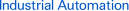# FAQ01906 of Push Buttons / Indicator Lamps FAQ

FAQ No. FAQ01906

Question

How do you calculate the control resistance for A3D/M2D Push Buttons LED lamps?

﻿

Set the control resistance within the range for the LED lamp characteristics.

(Set the forward current for the LED lamp to 8 mA min.)

Use the following formula to find the external resistance.

R = (E - VF)/IF (Ω)

E: Operating voltage (V)
VF: LED forward voltage (V)
IF: LED forward current (A)

Control Resistance (Recommended Values)

 Voltage Red Yellow (White) Green 5 VDC 165 Ω 140 Ω 145 Ω 12 VDC 515 Ω 490 Ω 495 Ω 24 VDC 1,100 Ω 1,090 Ω 1,095 Ω

Calculation Example: A3D

LED lamp illuminating color: Red

E = 24V
IF = 20mA
Ta = 25℃

Based on the VF-IF characteristics in the diagram below (red):
VF = 1.7 V when IF = 20 mA.

Therefore, inserting the values into the formula above (R = E - VF/IF(Ω)):
R = (24 (V) - 1.7 (V))/0.02 (A)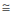1100 (Ω).

The recommended resistance is 1.1 kΩ at 1 W (2 x IF2R).

Note:Approximately twice this value is appropriate to provide a margin in the capacity of the resistor.

LED Characteristics (VF-IF Characteristics)

A3D Series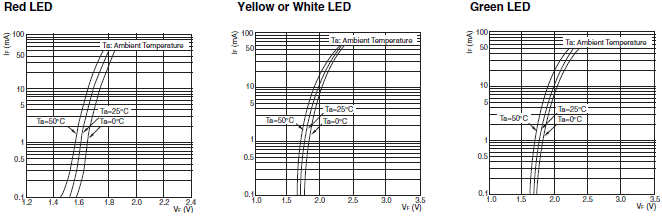A3A Series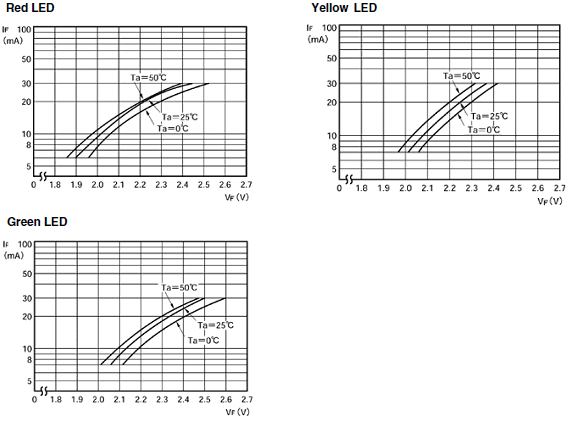Forward Current Reduction Curve

A3D Series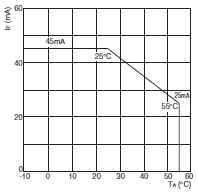Applicable models: A3D Push Buttons, A3A Push Buttons, etc.

## Recommended Products

###61F-G[]N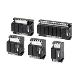Improved Design for a More Lightweight Construction and Reduced Standby Power Consumption.

###Z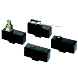Best-selling Basic Switch Boasting High Precision and Wide Variety

###WL, WLM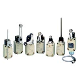Wide Range of Two-circuit Switches; Select One for the Operating Environment/Application